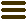The Software Design Metrics tool for the UMLParsing UML Diagram Information from Altova UModel XMI Files

June 20, 2012, Jürgen Wüst. Category: Tips & Tricks

I may have mentioned it before on this blog, but XMI interchange of UML models is notoriously difficult in practice. A major problem of model interchange concerns diagram information: what diagrams are there, which elements appear on the diagrams, and where are they located on the diagram? While the UML model itself often can be exchanged more or less intact between tools, the diagram information usually is lost in the process.

The UML defines a diagram interchange standard (UML DI), but it is not widely used. The standard mandates a very detailed description of UML diagrams. For instance, to show some class ‘Foo’ on a class diagram, it does not only specify to draw its class symbol at certain coordinates, but also whether to draw the operation or attribute compartments, and which operations and attributes should be visible in the compartments. After all, designers can choose to only show selected operations or attributes on a given diagram. Even every single operation parameter on a class diagram is linked to its parameter and type objects in the model.

Instead of UML DI, tool vendors often use proprietary XMI extensions to store the diagram information. Of course, these extensions are not understood by other tools, which explains why the diagrams are lost during model exchange. As a typical example of such an XMI extension, the following excerpt from an XMI file shows how Altova UModel saves its diagrams (I have replaced the XMI IDs for readability):

<xmi:XMI ... xmi:version="2.1">
<xmi:Documentation ... exporter="Altova UModel2012r2" exporterVersion="10"/>
<xmi:Extension extender="UModel">
<Diagrams>
<uml:RootElement xmi:id="E59RK7MUY3Y4">
<guiRootGuiDiagram xmi:type="uml:ClassDiagram" xmi:id="diag1" name="ClassDiagram" ...>
<nodeRect Left="210" Top="150" Right="271" Bottom="192"/>
<pos X="216" Y="119"/>
...
...
</guiRootGuiDiagram>
</uml:RootElement>
</Diagrams>
</xmi:Extension>
<uml:Model xmi:id="mdl1" name="Root">
<packagedElement xmi:type="uml:Class" xmi:id="cls1" name="Subclass">
<generalization xmi:type="uml:Generalization" xmi:id="gen1" general="cls2"/>
</packagedElement>
...
</uml:Model>
</xmi:XMI>

As we can see, the entire diagram information is contained within an <xmi:Extension> element. Altova UModel defines a new meta class “RootElement” in the UML namespace, with a composition association “guiRootGuiDiagram” that contains the diagrams of the model. A diagram in turn has a composition association “guiDiagramGuiLink” that contains all elements on the diagram. These are instances of meta classes “NodeLink” or “LineLink”. Each diagram element has a reference “guiLink_Element” to the model element it represents.

Out of the box, SDMetrics does not parse Altova UModel’s diagram information. But with the above information, we can extend SDMetrics’ XMI import facility. Simply add the following lines to the XMI transformation file:

<xmitransformation modelelement="diagram" xmipattern="guiRootGuiDiagram" recurse="true">
<trigger name="name" type="attrval" attr="name" />
<trigger name="type" type="attrval" attr="xmi:type" />
</xmitransformation>

</xmitransformation>

This maps SDMetrics’ “diagram” and “diagramelement” metaclasses for diagram information to UModel’s “guiRootGuiDiagram” and “guiDiagramGuiLink” elements, respectively.

So far, so good. Now let’s assume we want to define design rules that pertain to diagram guidelines such as “place subclasses below their superclass”. For that we need to check the coordinates of the diagram elements in the diagram. For connectors between elements such as associations or generalizations, it is also useful to know which diagram elements are attached to the connector.

In the XMI file above, the XML element <nodeRect Left="210" Top="150" Right="271" Bottom="192"/> provides the coordinates of a diagram node link. For line links, the XML attributes “guiLineLink_LineBegin” and “guiLineLink_LineEnd” reference the attached diagram node links. To extract this data from the XMI file, we extend SDMetrics’ meta model for diagram information by adding several attributes to the “diagramelement” type in the meta model definition file:

<modelelement name="diagramelement" >
<attribute name="element" type="ref">The element that is shown on the diagram.</attribute>
<attribute name="top" type="data">Top coordinate of a node link.</attribute>
<attribute name="left" type="data">Left coordinate of a node link.</attribute>
<attribute name="right" type="data">Right coordinate of a node link.</attribute>
<attribute name="bottom" type="data">Bottom coordinate of a node link.</attribute>
</modelelement>

The complete XMI transformations for the extended diagram elements look like this: